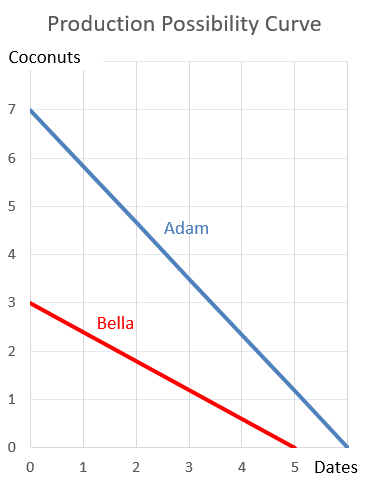# Fight Finance

#### CoursesTagsRandomAllRecentScores

The required return of a project is 10%, given as an effective annual rate. Assume that the cash flows shown in the table are paid all at once at the given point in time.

What is the Net Present Value (NPV) of the project?

 Project Cash Flows Time (yrs) Cash flow ($) 0 -100 1 0 2 121 When using the dividend discount model to price a stock: $$p_{0} = \frac{d_1}{r - g}$$ The growth rate of dividends (g): You want to buy an apartment priced at$500,000. You have saved a deposit of $50,000. The bank has agreed to lend you the$450,000 as an interest only loan with a term of 30 years. The interest rate is 6% pa and is not expected to change. What will be your monthly payments?

Your main expense is fuel for your car which costs $100 per month. You just refueled, so you won't need any more fuel for another month (first payment at t=1 month). You have$2,500 in a bank account which pays interest at a rate of 6% pa, payable monthly. Interest rates are not expected to change.

Assuming that you have no income, in how many months time will you not have enough money to fully refuel your car?

How is the AUD normally quoted in Australia? Using or terms?

The hardest and most important aspect of business project valuation is the estimation of the:

Calculate the price of a newly issued ten year bond with a face value of $100, a yield of 8% pa and a fixed coupon rate of 6% pa, paid semi-annually. So there are two coupons per year, paid in arrears every six months. A firm wishes to raise$50 million now. They will issue 5% pa semi-annual coupon bonds that will mature in 10 years and have a face value of \$100 each. Bond yields are 5% pa, given as an APR compounding every 6 months, and the yield curve is flat.

How many bonds should the firm issue?

Adam and Bella are the only people on a remote island. Their production possibility curves are shown in the graph.Which of the following statements is NOT correct?

Which of the following statements about vanilla floating coupon bonds is NOT correct? A vanilla floating coupon bond's: